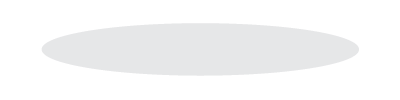# Chapter 8: Costs, Production, Supply

1. Basics
1. Law of Diminishing Marginal Productivity or Returns
2. Relationship among Average and Marginal Cost and Product Curves
3. Economies of Scale
4. Relationships
5. Short and Long Run Cost Curves
6. Costs
2. Production
1. Short Run vs. Long Run
1. Law of Diminishing Marginal Productivity or Returns
1. a short run phenomenon
2. at first, production increases at an increasing rate
1. MPPs becoming increasingly larger
2. MPPs- marginal physical products
3. then it increases at a decreasing rate, and eventually decreases
2. ​​Relationship to Costs
1. Average physical product
1. ​APP = (total output) / (variable input)
2. Marginal physical product
1. ​MPP = (change in output) / (change in input)
3. The relationship of MPP to APP is the converse of the relationship of MC to AVC
1. both:
1. as the marginal is less than the average, the average is declining
2. MPP intersects APP at APP maximum point
3. MC intersects AVC at AVC minimum point
3. Costs
1. Fixed Costs
1. In total, they do not vary with changes in output
2. At zero output:
1. total costs = total fixed costs
2. total fixed costs
1. ​examples: depreciation costs, property taxes, salaries
3. ​​Average fixed costs (AFC)
1. total fixed costs divided by output
2. AFC = TFC ± Q
3. decline as output increases
2. ​​Variable Costs
1. In total, vary as output changes
2. Include:
1. changes in number of employees
2. energy costs related to production changes
3. travel expenses
3. Average variable costs
1. AVC = TVC / Q
2. TVC – total variable costs
3. Q – output level
4. Tend to initially decrease, hit a minimum, then increase
3. Total Costs
1. ​Equation
1. TC = TFC + TVC
2. TC – total costs
3. TFC – total fixed costs
4. TVC – total variable costs
2. ​​​Average total costs (ATC)
1. ATC = AFC + AVC
3. MC intersects both the AVC curve and the ATC curve at their minimum points
4. ​​​Economies of Scale
1. Those savings in average costs of production per unit that are realized as the firm increases production of goods
5. Value of a Large-Size Firm
1. Can higher specialists, which will increase efficiency
2. The increase in volume of output allows ATC to decrease
6. Profits
1. Economic profits
1. income or revenue minus explicit and implicit costs
2. Accounting profits
1. income or revenue minus explicit costsYou just finished Chapter 8: Costs, Production, Supply. Nice work!

Tip: Use ← → keys to navigate!

### How to cite this note (MLA)

Aboukhadijeh, Feross. "Chapter 8: Costs, Production, Supply" StudyNotes.org. Study Notes, LLC., 12 Oct. 2013. Web. 29 Sep. 2023. <https://www.apstudynotes.org/microeconomics/outlines/chapter-8-costs-production-supply/>.